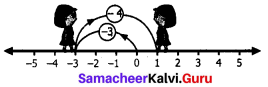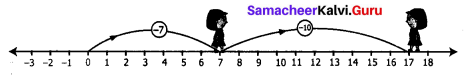# Samacheer Kalvi 7th Maths Solutions Term 1 Chapter 1 Number System Ex 1.2

Students can Download Maths Chapter 1 Number System Ex 1.2 Questions and Answers, Notes Pdf, Samacheer Kalvi 7th Maths Book Solutions Guide Pdf helps you to revise the complete Tamilnadu State Board New Syllabus and score more marks in your examinations.

## Tamilnadu Samacheer Kalvi 7th Maths Solutions Term 1 Chapter 1 Number System Ex 1.2

Question 1.
Fill in the blanks
(i) -44 + ____ = -88
(ii) ___ – 75 = -45
(iii) ___ – (+50) = -80
Solution:
(i) -44
(ii) 30
(iii) -30

Question 2.
Say True or False.
(i) (-675) – (-400) = -1075
(ii) 15 – (-18) is the same as 15 + 18
(iii) (-45) – (-8) = (-8) – (-45)
Solution:
(i) False
(ii) True
(iii) False

Question 3.
Find the value of the following.
(i) -3 – (-4) using number line.
Solution:
We start at zero facing positive direction. Move 3 units backward to represent (-3). Then turn towards the negative side and move 4 units backwards. We reach+1.∴ (-3) – (-4) = +1

(ii) 7 – (-10) using number line
Solution:We start at zero facing positive direction. Move 7 units forward to represent (+7). Then turn towards the negative side and move 10 units backwards.
We reach +17
∴ 1 – (-10) = +17

(iii) 35 – (-64)
Solution:
35 – (-64) = 35+ (Additive inverse of-64) = 35 + (+64) = 99
∴ 35 – (-64) = 99

(iv) -200 – (+100)
Solution:
-200 – (+100) = -200 + (Additive inverse of+100) = -200 + (-100) = -300
-200 – (+100) = -300Question 4.
Kabilan was having 10 pencils with him. He gave 2 pencils to senthil and 3 to Karthick. Next day his father gave him 6 more pencils, from that he gave 8 to his sister. How many pencils are left with him?
Solution:
Total pencils Kabilan had = 10
No. of pencils given to Senthil = 2
No. of pencils given to Karthick = 3.
Now number of pencils left with Kabilan = 10 – 2 – 3 = 8 – 3 = 5
Number of pencils got from his father = 6
No. total pencils Kabilan had = 5 + 6 = 11
Number of pencils given to his sister = 8
Number of pencils left with Kabilan = 11 – 8 = 3

Question 5.
A lift is on the ground floor. If it goes 5 floors down and then moves up to 10 floors from there, then in which floor will the lift be?
Solution:
Initially the lift will be in the ground floor representing ‘0’
It goes to 5 floors down ⇒ -5
Then it moves 10 floors up +10.
Now the lift will be = 0 – 5 + 10 = -5 + 10
= 5th floor (above the ground floor)

Question 6.
When Kala woke up, her body temperature was 102°F. She took medicine for fever. After 2 hours it was 2°F lower. What was her temperature then?
Solution:
Kala’s temperature initially = 102°F
After two hours the temperature decreased = -2°F
Now the final temperature = 102°F – 2°F = 100°F

Question 7.
What number should be added to (-17) to get -19?
Solution:
According to the problem = -17 + A number = -19
The number = -19 + 17 = -2
∴ -2 should be added to -17 to get -19Question 8.
A student was asked to subtract (-12) from -47. He got -30. Is he correct? Justify.
Solution:
Subtracting -12 from -47, we get
-47 – (-12) = -47 + (Additive inverse of-12)
= -47 + (+12) = -35
But the students answer is -30.
So he is not correct.

Objective Type Questions

Question 9.
(-5) – (-18)
(i) 23
(ii) -13
(iii) 13
(iv) -23
Solution:
(iii) 131

Question 10.
(-100) – 0 + 100 =
(i) 200
(ii) 0
(iii) 100
(iv)-200
Solution:
(ii) 0